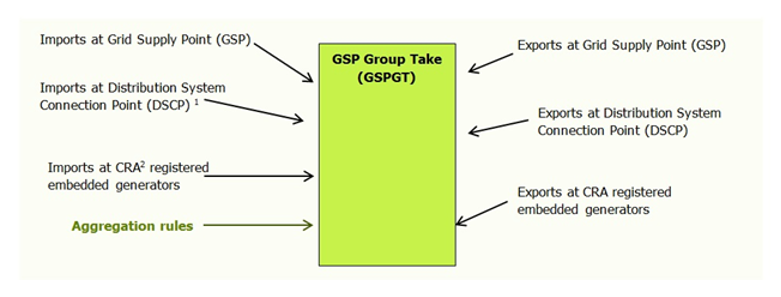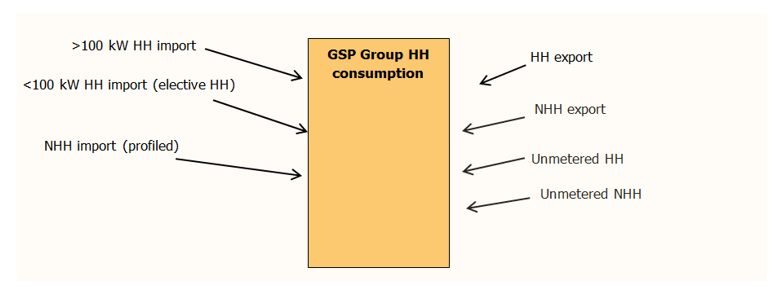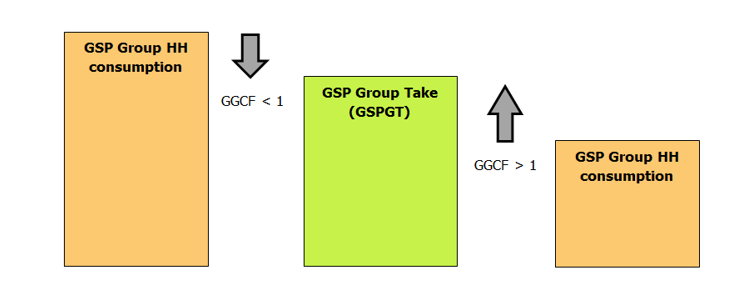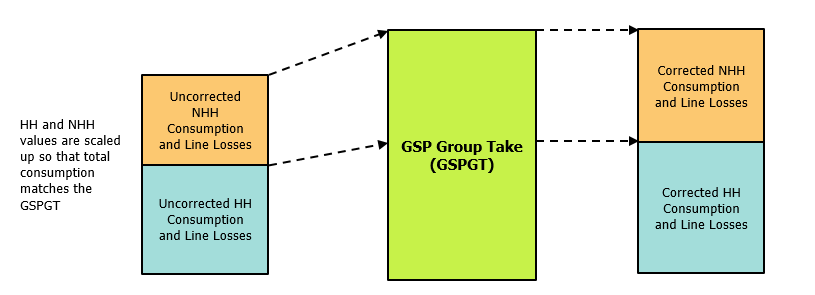# Grid Supply Point (GSP) Group Correction

v 6.0
 Grid Supply Point (GSP) Group Correction Guidance Note

## What is GSP Group Take?

GSP Group Take is the net energy measured going from/to a particular Local Distribution System (i.e. a GSP Group) in a Settlement period. Theoretically, if we were to add up all the estimated and actual meter readings in a single GSP Group for a particular Settlement period, then the total metered volume should equal the GSP Group Take. However, this tends not to be the case due to the over or under accounting of energy for a number of reasons, including:

• Inaccuracies arising from the use of Profiles to allocate Non Half Hourly (NHH) metered volumes to a particular Settlement period;

• Errors and approximations in the calculation of Line Loss Factors;

• Metering system errors and meters that have the incorrect energisation status;

• Incorrect actual or estimated Meter Advances;

• Undetected theft and detected theft not settled; and

• Errors related to Unmetered Supplies (UMS).

GSP Group Take

This is the GSP Group Take 1,2 based on actual meter readings gathered by the Central Data Collection Agent (CDCA)Supplier Volume Allocation (SVA) energy## What is GSP Group Correction?

GSP Group Correction is the mechanism that allocates the total error in metered volumes in each GSP Group between Suppliers. GSP Group Correction essentially addresses the under or over accounting of energy by applying a correction factor to Suppliers’ SVA energy such that the aggregate energy allocated to Suppliers is equal to the GSP Group Take in each Settlement period. As a consequence, issues with the quality of SVA data can impact the energy allocated to all Suppliers.

## What are GSP Group Correction Factors?

GSP Group Correction Factors (GGCFs) are the correction factors used to ensure that the total energy allocated to Suppliers in each Settlement period in each GSP Group matches the energy entering the GSP Groups from the transmission system, adjoining GSP Groups and through embedded generation.GSP Group Correction Factor values of less than one (i.e. the scaling down of energy) may result from:

• the over-accounting of import energy in SVA;

• the under-accounting of export energy in SVA; or

• under-accounting of Grid Supply Point metering.

GSP Group Correction Factor values of greater than one (i.e. the scaling up of energy) may result from:

• the under-accounting of import energy in SVA;

• the over-accounting of export energy in SVA; or

• over-accounting of GSP metering.

## How are GSP Group Correction Factors calculated?

The GSP Group Correction Factor (GCF) for each Settlement period and GSP Group is calculated in accordance with Section S: Annex S-2 of the BSC as follows:

GCFHj = 1 + (GSPGTHj - ΣN GCHNj) / (ΣN (GCHNj * WTN))

where:

• H = GSP Group

• j = Settlement period

• N = Consumption Component Class (CCC)

• WTN = GSP Group Correction Scaling Weight

• GSPGTHj = GSP Group Take

• GCHNj = GSP Group Half Hourly Consumption, and is calculated as:

GCHNj = ΣzCHZNj + ΣZ CLOSSHZNj

Where:

• Z = Supplier

• CHZNj = Half Hourly Consumption (Non Losses)

• CLOSSHZNj = Half Hourly Consumption (Losses)

What is a Consumption Component Class (CCC)?

There are 62 CCCs defined in Annex X-2 of the BSC. All SVA Energy is allocated to one of these CCCs depending on its attributes in the following areas:

• Is the energy Active Import (AI-consumption) or Active Export (AE-generation)?

• Is the Data Aggregator Half Hourly (HH) or Non Half Hourly (NHH)?

• Is the energy basic consumption/generation (C), metering system specific line losses (M) or metering system non-specific line losses (L)?

• Is the energy volume an actual (A) or estimated (E) value?

• Is the Metering System in Measurement Class3 ‘A’, ‘B’, ‘C’, ‘D’, ‘E’, ‘F’ or ‘G’?

## How are GSP Group Correction Factors applied?

With regards to the application of GSP Group Correction, is it applied to all SVA metered volumes? The answer is no. GSP Group Correction is not applied to all SVA metered volumes. The GSP Group Correction Factor calculation refers to a Scaling Weight for each Consumption Component Class (CCC), which defines how much GSP Group Correction should be applied to that CCC (relative to the others).

These Scaling Weights have been set to:

• for all AE CCCs and HH AI CCCs with Measurement Class ‘C’, ‘D’ and ‘E’;

• for AI CCCs with a consumption component indicator of ‘C’ and Measurement Class ‘A’, ‘B’, ‘F’ and ‘G’; and

• 1.2 for AI CCCs with a consumption component indicator of ‘L’ or ‘M’ and measurement class ‘A’, ‘B’, ‘F’ and ‘G’.

The following diagram provides an example of the current application of GSP Group Correction.The application of GSP Group Correction is not uniform. For example, with a Scaling Weight of 1.2, Non Half Hourly Line Losses receive a greater share of the GSP Group Correction than Non Half Hourly Consumption, which has a Scaling Weight of 1.0.

## How does GSP Group Correction affect (small or bigger) Suppliers?

The target limit for GSP Group Correction Factors is ±10% from 1. However, there are many cases when Group Correction Factors are higher than 1.1 or lower than 0.9 and these outliers are often attributed to profile coefficients that give shape to the Non Half Hourly energy, temperature effects, microgeneration (mainly photovoltaics) embedded in certain GSP Groups (mainly Eastern and South Western), high wind generation (mainly in Scotland), bank holidays, etc.

The graph we publish in the Trading Operations Report shows the distribution of Group Correction Factors with the majority of them correcting Non Half Hourly volumes between 0 and ±10% for all Suppliers. However, we do get some occasional data issues which are usually resolved before Final Reconciliation (RF).

The same Group Correction Factor is applied to all Suppliers’ energy volumes within each GSP Group. There is no differentiation between small or big Suppliers according to their market and energy share. Some potential issues small Suppliers might have are smaller forecasting teams and a lack of understanding/experience, which makes their hedging decisions more difficult.

## Where can I find the GSP Group Correction Factors and Scaling Weights?

The GSP Group Correction Factors for the latest Settlement run for each Settlement day can be found in the GSP Group Correction Factors page of the Elexon Portal.

The GSP Group Correction Scaling Weights can be found in the ‘GSP Group Correction Scaling Factor’ table of Market Domain Data (MDD), on the MDD page of the Elexon Portal.